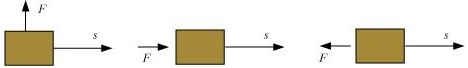Worksheet Solutions: Work and Energy

# Worksheet Solutions: Work and Energy - Science Class 9

Q.1. Fill in the blank

(i) When a ball is thrown upwards, kinetic energy is transformed into potential energy.
(ii) The sum of the potential and kinetic energies of a body is called mechanical energy.
(iii) Work is measured as a product of force and distance.
(iv) The electricity meter installed in our home measures electric energy in the units of kWh.
(v) The work done on a body moving in a circular path is zero.

Q.2. How are Joule (J) and ergs (erg) related?
(a) 1J = 107erg
(b) 1erg = 107J
(c) 1J = 10-7erg
(d) None

Q.3.  If the force is applied at an angle θ then work done is
(a) W = FS Cos θ  F = Force
(b) W = FS θ S =  Distance
(c) W = FS Sin  θ W = work
(d) None

Q.4. If a body is stored at a height ‘h’ then it will posses:
(a) Kinetic energy
(b) Potential energy
(c) Both
(d) None

Q.5. If the body starts from rest, then change in its kinetic energy is
(a) Positive
(b) Negative
(c) Zero
(d) May be Positive or negative depending upon the mass of the body

Q.6. When do we say that work is done?

Work is done when a force acts on an object and displacement occurs in the direction of force.

Q.7. Define 1 J of work.

1 J is that work which is done when a force of 1 N is applied on an object and it moves a distance of 1 m in the direction of force.

Q.8. What is the kinetic energy of an object?

Kinetic energy of an object is a kind of mechanical energy and it is present in that object due to its state of motion(movement).

Q.9. What is power?

Power is the rate of work done. It is denoted by P.
power = work/time
P = W / t

Q.10. An object thrown at a certain angle to the ground moves in a curved path and falls back to the ground. The initial and the final points of the path of the object lie on the same horizontal line. What is the work done by the force of gravity on the object?

For calculating work done there must be displacement but since in this example vertical displacement is zero (as initial and final points lie on the same horizontal line) so the work done by force of gravity is zero.

Q.11. Illustrate the law of conservation of energy by discussing the energy changes which occur when we draw a pendulum bob to one side and allow it to oscillate. Why does the bob eventually come to rest? What happens to its energy eventually? Is it a violation of the law of conservation of energy?

The bob eventually comes to rest due to the frictional force offered by the air and the rigid support holding the thread.
It is not a violation of the law of conservation of energy since mechanical energy can get converted into another form of energy which cannot be utilised for useful work. This loss of energy is called dissipation of energy.

Q.12. Define average power.

An agent may not be able to perform same amount of work in a given period of time always. In other words power of that agent may vary with time. Hence we can take average power in such situations. Average power is defined as average amount of work done by a body per unit time (i.e. total energy consumed divided by total time).

Q.13.  In each of the following a force, F is acting on an object of mass, m. The direction of displacement is from west to east shown by the longer arrow. Observe the diagrams carefully and state whether the work done by the force is negative, positive or zero.• Since in this diagram displacement is perpendicular to the direction of force, so work done is zero.
• Since in this diagram displacement is in the direction of force, so work done is positive.
• Since in this diagram displacement is in the opposite direction of the force applied hence work done is negative.

Q.14. Define 1 watt of power.

If an agent works at the rate of 1 J/s then the power of that agent is 1 watt (where watt is the unit of power).

Q.15. A battery lights a bulb. Describe the energy changes involved in the process.

Chemical energy of battery is changed into heat energy and light energy.

The document Worksheet Solutions: Work and Energy | Science Class 9 is a part of the Class 9 Course Science Class 9.
All you need of Class 9 at this link: Class 9

## Science Class 9

66 videos|355 docs|97 tests

## Science Class 9

66 videos|355 docs|97 testsExplore Courses for Class 9 examSignup to see your scores go up within 7 days! Learn & Practice with 1000+ FREE Notes, Videos & Tests.
10M+ students study on EduRev
Track your progress, build streaks, highlight & save important lessons and more!
Related Searches

,

,

,

,

,

,

,

,

,

,

,

,

,

,

,

,

,

,

,

,

,

;